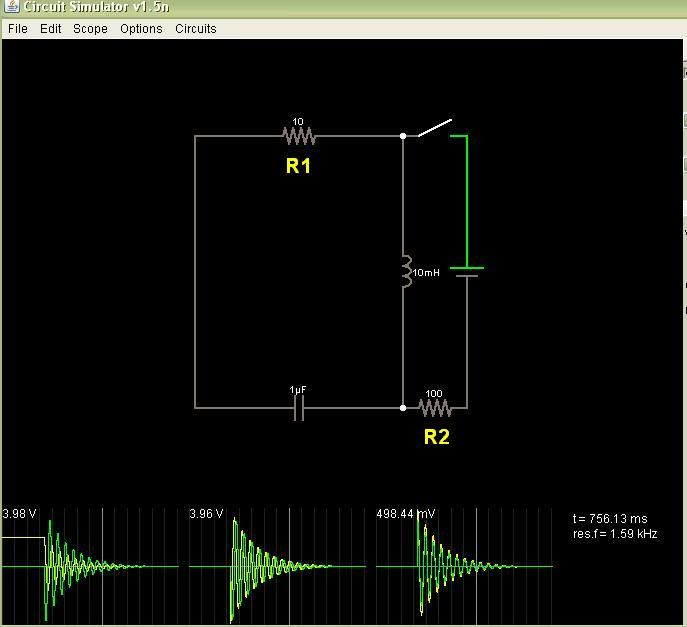# Voltage drop in oscillating circuit

here is the circuit I am studying:I am using fastead's online Java circuit simulator to design it before I commit to assembling the thing.

One thing I found puzzling, if I change R2 resistor from 50 Ohm to 100 Ohm, voltage in the circuit drops from 7.96V to 3.98V. I thought resistor R2 doesn't count at all, since it is in the open part of the circuit. But actually it seems that R2 is the major player.

Can somebody explain this?

Is it possible to have 2 switches which open at once and completely cut off the R2 and DC voltage source?

R2 is only important when the switch is closed, when it is open there is no current flowing through it and it has no effect. However when the switch is closed that resistor limits the rise time of the current into the system. If you are closing the switch for a fixed amount of time then yes the voltage will drop because it will not have had sufficient time to fully charge up. Try increasing the switch closed time and you will see the initial voltage rise again.

Yeah, I had a different explanation: current is changing direction through the inductor. One moment it is going in one direction next moment in the opposite. And there is still Ground terminal of the original voltage source. So what happens, when current goes in the direction of R1 nothing happens, but when current goes in a direction of the capacitor and R2, than it splits and some of current goes through R2 into the ground on the original voltage source.

Can that be possible explanation?

Can that be possible explanation?

No.

but when current goes in a direction of the capacitor and R2, than it splits and some of current goes through R2 into the ground on the original voltage source.

There is no path for current through R2 once the switch is open. You need a complete path for any current to flow, that is fundamental physics.

I understand that, but there is the energy of the magnetic field, stored in the inductor. Inductor is more or less acting as an oscillating battery. Path is closed, since one part of current flows into the earth terminal.

Path is closed, since one part of current flows into the earth terminal.

No there is no earth terminal in that circuit. R2 takes no part in the current flow once the switch is open. It is only connected to one end of the battery and the other end is going nowhere.

My suspicion is that the voltage mentioned is the amplitude of the oscillations. When the switch is closed for a while the inductor is taking a fixed current inversely proportional to R2. Thus its stored energy is inversely proportional to R2 squared (energy = 0.5LI^2)

Upon opening the switch this energy drives the L-C-R1 resonant circuit whose amplitude of initial oscillation is proportional to the square root of initial energy. R1 damps the oscillations and the smaller it is the less it damps.

When the switch is open R2 plays no part but it does set the initial conditions.

That is good explanation. With 100 Ohm resistor one is putting less energy into the inductor, than with 50 Ohm. That is why voltage is lower with R2= 100 Ohm than R2= 50 Ohm.

When you close the switch, eventually the time-dependent behavior of the components ends ("step response".) The capacitor acts like an open circuit, the inductor acts like a short, and you're left with a simple voltage divider consisting of R1, R2, and the power source. So the initial voltage on the cap, and current in the inductor, are going to be dependent on the ratio of the resistors. And thus the amplitude to the oscillations when you move the switch as well.

If you close the switch for less time than it takes to reach a steady state, the resistors will still be acting as a voltage divider...

Job done. Thank you guys. You all brought me to understanding it well.

I had to study this circuit carefully, because I was worried that inductor might blow up oscillator, with spike, when I put it in a circuit.

westfw:
When you close the switch, eventually the time-dependent behavior of the components ends (“step response”.) The capacitor acts like an open circuit, the inductor acts like a short, and you’re left with a simple voltage divider consisting of R1, R2, and the power source. So the initial voltage on the cap, and current in the inductor, are going to be dependent on the ratio of the resistors. And thus the amplitude to the oscillations when you move the switch as well.

If you close the switch for less time than it takes to reach a steady state, the resistors will still be acting as a voltage divider…

Just to set thie record straight R1 / R2 do not act as a voltage divider in the steady state (nor at any other time as they are not connected to each other directly!). In the steady state R1 is open circuit (via the capacitor). When the switch is closed (steady state) only the battery voltage V and R2 set the current in the inductor: I = V/R2

In the steady state both the capacitor and the inductor have 0V across them - they only see voltage during the switching transients.